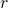# Geometric Sequences: Development

On the page, Geometric Sequences: Introduction we read that a geometric sequence can be defined using the first term and the common ratio.

On this page, we look for the general term for a geometric sequence.

On the following applet, you are given the first term and the common ratio for ten different geometric progressions. You are then asked to find one of the terms in the sequence.

Generalise your calculations usingto represent the term you need to calculate,to represent the first term, andto represent the ratio.

Now the general term for arithmetic sequence can be calculated either by using one term and the common difference; or by using two terms. Similarly, we can calculate the general term of the geometric progression using one term and the common ratio; or by using two terms.

## Short Comprehension Exercise:

Find the general term for each of the ten sequences in the applet above. (Use the refresh icon to show the first sequence).

## More Difficult

On the following applet, you are presented with two terms of a geometric sequence. Calculate the general term for the sequence.

I’m done! Take me back to the Sequences and Series Menu!